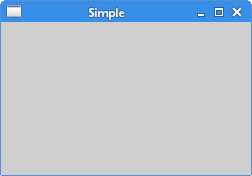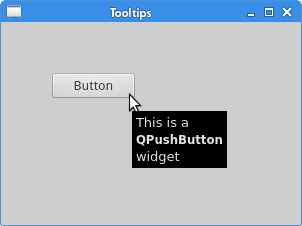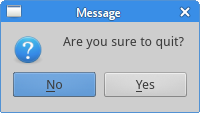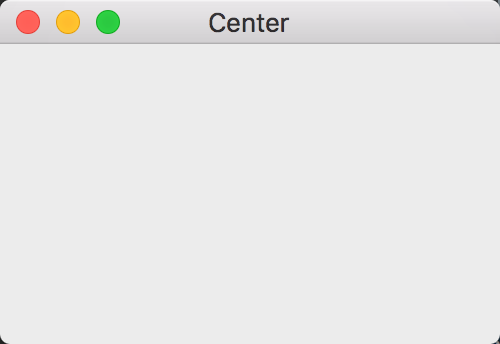# Hello World

## 例1，简单的窗口

``````#!/usr/bin/python3
# -*- coding: utf-8 -*-

"""
ZetCode PyQt5 tutorial

In this example, we create a simple
window in PyQt5.

author: Jan Bodnar
website: zetcode.com
Last edited: August 2017
"""

import sys
from PyQt5.QtWidgets import QApplication, QWidget

if __name__ == '__main__':

app = QApplication(sys.argv)

w = QWidget()
w.resize(250, 150)
w.move(300, 300)
w.setWindowTitle('Simple')
w.show()

sys.exit(app.exec_())
``````

``````import sys
from PyQt5.QtWidgets import QApplication, QWidget
``````

``````app = QApplication(sys.argv)
``````

``````w = QWidget()
``````

QWidge控件是一个用户界面的基本控件，它提供了基本的应用构造器。默认情况下，构造器是没有父级的，没有父级的构造器被称为窗口（window）。

``````w.resize(250, 150)
``````

resize()方法能改变控件的大小，这里的意思是窗口宽250px，高150px。

``````w.move(300, 300)
``````

move()是修改控件位置的的方法。它把控件放置到屏幕坐标的(300, 300)的位置。注：屏幕坐标系的原点是屏幕的左上角。

``````w.setWindowTitle('Simple')
``````

``````w.show()
``````

show()能让控件在桌面上显示出来。控件在内存里创建，之后才能在显示器上显示出来。

``````sys.exit(app.exec_())
``````

`exec_()`之所以有个下划线，是因为`exec`是一个Python的关键字。## 例2，带窗口图标

``````#!/usr/bin/python3
# -*- coding: utf-8 -*-

"""
ZetCode PyQt5 tutorial

This example shows an icon
in the titlebar of the window.

Author: Jan Bodnar
Website: zetcode.com
Last edited: August 2017
"""

import sys
from PyQt5.QtWidgets import QApplication, QWidget
from PyQt5.QtGui import QIcon

class Example(QWidget):

def __init__(self):
super().__init__()

self.initUI()

def initUI(self):

self.setGeometry(300, 300, 300, 220)
self.setWindowTitle('Icon')
self.setWindowIcon(QIcon('web.png'))

self.show()

if __name__ == '__main__':

app = QApplication(sys.argv)
ex = Example()
sys.exit(app.exec_())
``````

``````class Example(QWidget):

def __init__(self):
super().__init__()
...
``````

``````self.initUI()
``````

``````# 自己准备一个web.png
self.setGeometry(300, 300, 300, 220)
self.setWindowTitle('Icon')
self.setWindowIcon(QIcon('web.png'))
``````

``````if __name__ == '__main__':

app = QApplication(sys.argv)
ex = Example()
sys.exit(app.exec_())
``````## 例3，提示框

``````#!/usr/bin/python3
# -*- coding: utf-8 -*-

"""
ZetCode PyQt5 tutorial

This example shows a tooltip on
a window and a button.

Author: Jan Bodnar
Website: zetcode.com
Last edited: August 2017
"""

import sys
from PyQt5.QtWidgets import (QWidget, QToolTip,
QPushButton, QApplication)
from PyQt5.QtGui import QFont

class Example(QWidget):

def __init__(self):
super().__init__()

self.initUI()

def initUI(self):

QToolTip.setFont(QFont('SansSerif', 10))

self.setToolTip('This is a <b>QWidget</b> widget')

btn = QPushButton('Button', self)
btn.setToolTip('This is a <b>QPushButton</b> widget')
btn.resize(btn.sizeHint())
btn.move(50, 50)

self.setGeometry(300, 300, 300, 200)
self.setWindowTitle('Tooltips')
self.show()

if __name__ == '__main__':

app = QApplication(sys.argv)
ex = Example()
sys.exit(app.exec_())
``````

``````QToolTip.setFont(QFont('SansSerif', 10))
``````

``````self.setToolTip('This is a <b>QWidget</b> widget')
``````

``````btn = QPushButton('Button', self)
btn.setToolTip('This is a <b>QPushButton</b> widget')
``````

``````btn.resize(btn.sizeHint())
btn.move(50, 50)
``````## 例4，关闭窗口

``````QPushButton(string text, QWidget parent = None)
``````

`text`参数是想要显示的按钮名称，`parent`参数是放在按钮上的组件，在我们的 例子里，这个参数是`QWidget`。应用中的组件都是一层一层（继承而来的？）的，在这个层里，大部分的组件都有自己的父级，没有父级的组件，是顶级的窗口。

``````#!/usr/bin/python3
# -*- coding: utf-8 -*-

"""
ZetCode PyQt5 tutorial

This program creates a quit
button. When we press the button,
the application terminates.

Author: Jan Bodnar
Website: zetcode.com
Last edited: August 2017
"""

import sys
from PyQt5.QtWidgets import QWidget, QPushButton, QApplication
from PyQt5.QtCore import QCoreApplication

class Example(QWidget):

def __init__(self):
super().__init__()

self.initUI()

def initUI(self):

qbtn = QPushButton('Quit', self)
qbtn.clicked.connect(QCoreApplication.instance().quit)
qbtn.resize(qbtn.sizeHint())
qbtn.move(50, 50)

self.setGeometry(300, 300, 250, 150)
self.setWindowTitle('Quit button')
self.show()

if __name__ == '__main__':

app = QApplication(sys.argv)
ex = Example()
sys.exit(app.exec_())
``````

``````from PyQt5.QtCore import QCoreApplication
``````

``````qbtn = QPushButton('Quit', self)
``````

``````qbtn.clicked.connect(QCoreApplication.instance().quit)
``````## 例5，消息盒子

``````#!/usr/bin/python3
# -*- coding: utf-8 -*-

"""
ZetCode PyQt5 tutorial

This program shows a confirmation
message box when we click on the close
button of the application window.

Author: Jan Bodnar
Website: zetcode.com
Last edited: August 2017
"""

import sys
from PyQt5.QtWidgets import QWidget, QMessageBox, QApplication

class Example(QWidget):

def __init__(self):
super().__init__()

self.initUI()

def initUI(self):

self.setGeometry(300, 300, 250, 150)
self.setWindowTitle('Message box')
self.show()

def closeEvent(self, event):

"Are you sure to quit?", QMessageBox.Yes |
QMessageBox.No, QMessageBox.No)

event.accept()
else:
event.ignore()

if __name__ == '__main__':

app = QApplication(sys.argv)
ex = Example()
sys.exit(app.exec_())
``````

``````reply = QMessageBox.question(self, 'Message',
"Are you sure to quit?", QMessageBox.Yes |
QMessageBox.No, QMessageBox.No)
``````

``````if reply == QMessageBox.Yes:
event.accept()
else:
event.ignore()
``````## 例6，窗口居中

``````#!/usr/bin/python3
# -*- coding: utf-8 -*-

"""
ZetCode PyQt5 tutorial

This program centers a window
on the screen.

Author: Jan Bodnar
Website: zetcode.com
Last edited: August 2017
"""

import sys
from PyQt5.QtWidgets import QWidget, QDesktopWidget, QApplication

class Example(QWidget):

def __init__(self):
super().__init__()

self.initUI()

def initUI(self):

self.resize(250, 150)
self.center()

self.setWindowTitle('Center')
self.show()

def center(self):

qr = self.frameGeometry()
cp = QDesktopWidget().availableGeometry().center()
qr.moveCenter(cp)
self.move(qr.topLeft())

if __name__ == '__main__':

app = QApplication(sys.argv)
ex = Example()
sys.exit(app.exec_())
``````

`QtGui.QDesktopWidget`提供了用户的桌面信息，包括屏幕的大小。

``````self.center()
``````

``````qr = self.frameGeometry()
``````

``````cp = QDesktopWidget().availableGeometry().center()
``````

``````qr.moveCenter(cp)
``````

``````self.move(qr.topLeft())
``````